American Journal of Modern Physics
Volume 5, Issue 2, March 2016, Pages: 20-24

Gravitational Waves, Newton’s Law and Coulomb's Law Interpreted by Particle Radiation and Interaction Theory Based on Yangton & Yington Theory

Edward T. H. Wu

Solar Buster Corporation, Los Angeles, United States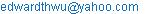Edward T. H. Wu. Gravitational Waves, Newton’s Law of Universal Gravitation and Coulomb's Law of Electrical Forces Interpreted by Particle Radiation and Interaction Theory Based on Yangton & Yington Theory. American Journal of Modern Physics. Vol. 5, No. 2, 2016, pp. 20-24. doi: 10.11648/j.ajmp.20160502.11

Received: March 4, 2016; Accepted: March 10, 2016; Published:March 30, 2016

Abstract: The structures of Photon, Higgs Boson, Electron, and Positron are proposed based on "Wu’s Particle", a Yangton and Yington circulating pair. Gravitational Force is generated by the attractive force through close contact between two Higgs Bosons of String Structures made of "Wu’s Particles" with the same circulation direction. Electrical Forces are formed by either attractive or repulsive forces via close contact between Electrons and Positions/Protons. Higgs Boson and Electron Radiations with Inverse Square Law and Contact Interactions, defined as Particle Radiation and Interaction Theory, are proposed as the mechanisms to explain Newton’s Law of Universal Gravitation and Coulomb’s Law of Electrical Forces. Furthermore, Gravitational Waves is the fluctuation of the total Gravitational Forces of two merging Black Holes, which can be interpreted by Higgs Boson Radiation and Interaction Theory based on Yangton & Yington Theory, as detected in Line of Sight by the Earth observers.

Keywords: Yangton, Yington, Photon, Wu’s Particle, Higgs Boson, Electron, Positron, Gravitational Force, Electrical Forces, Higgs Boson Radiation, Electron Radiation, Particle Radiation and Interaction, Newton’s Law, Coulomb’s Law, Gravitational Waves

1. Introduction

It is proposed by Edward T. H. Wu, a hypothetical theory  that a pair of super fine particles, Yangton and Yington, with an inter-attractive "Force of Creation", can be spontaneously created at anywhere and anytime in the universe as the "Origin of Creation" that could make "Something from Nothing". Meantime, because of the reversible process enforced by the inter-attractive "Force of Creation", after formation, within no time, Yangton and Yington recombine themselves to destroy each other so as to ensure that "Something Becomes Nothing" and the whole universe returns back to an empty space.

In the beginning, there was nothing in the universe until the Big Bang  explosion. It is proposed that Photon  was first formed by a pair of Yangton and Yington particles. Instead of recombination and destroy each other, triggered by the Big Bang explosion, they started moving against each other permanently in a circular orbit which is known as "Wu’s Particle". A free "Wu’s Particle" can travel in space at light speed known as "Photon" which can combine Particle Physics  and Quantum Mechanics  to explain a variety of properties of light . The still Wu’s Particle on the other hand forms the building block of all matters such as those Subatomic Particles including Quarks, Neutrinos, Higgs Bosons, Electrons, Positrons, Protons, Neutrons and Dark Matters .

Furthermore, it is assumed that "Force of Creation" is the only fundamental force in the universe that can create and unify the Four Basic Forces . Gravitational Force  is induced by the attractive force through close contact between two Higgs Bosons with String Structures  that are made of "Wu’s Particle" having the same circulation direction. Electric Forces , either attractive or repulsive, are formed via close contact between static Electrons and Protons/Positrons. Electromagnetic Force  however is generated between moving electrons and positrons/Protons. Two atoms both with single outer layer electrons, can form attractive force between each other while having electron circulating in the same direction and repulsive force while in the opposite directions, and this is known as magnetic forces .

Although Newton’s Law of Universal Gravitation  and Coulomb’s Law of Electrical Forces  are known and accepted by scientists for centuries, how those forces work and how they travel and propagate in space were never fully understood. Recently Gravitational Waves from a pair of merging black holes were detected by LIGO Lab  which has increased tremendous attentions on the subject. In this paper, Particle Radiations and Interaction theory are proposed as the mechanisms to explain these phenomena based on Yangton and Yington theory.

2. Yangton and Yington Circulating Pair

It is proposed that a pair of super fine particles, Yangton and Yington with an inter-attractive "Force of Creation" (Fig. 1), can be spontaneously formed in the universe at anytime and anywhere. However, due to the enforcement of the inter-attractive "Force of Creation", Yangton and Yington immediately recombine and destroy each other such that something becomes nothing again and everything disappeared in the empty space.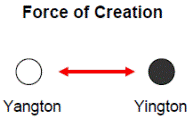Fig. 1. A Yangton and YIngton Pair and Force of Creation.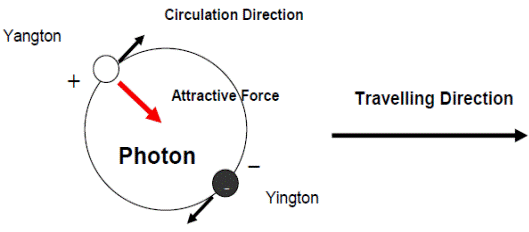Fig. 2. A hypothetical Photon.

Not until the Big Bang explosion, Yangton and Yington pair became permanently exist by absorbing "Energy of Formation" and started moving simultaneously after each other in a circular orbit balanced by the centrifugal force against the inter-attractive "Force of Creation" (Fig. 2). A free Yangton and Yington circulating pair known as Photon can travel in different Matters at different speeds. A still Yangton and Yington circulating pair known as "Wu’s Particle", on the other hand becomes the basic building block of all Matters.

2.1. Wu’s Particle

It is assumed that "Wu’s Particle", a still Yangton and Yington circulating pair is confined inside the substance to form all matters in the universe. With the preference of orientation due to the attractive force between Yangton and Yington particles or the dipoles in the adjacent "Wu’s Particles", unique subatomic particle structures can be formed by using "Wu’s Particle" as the building block (Fig. 3).

2.2. String Structures

String and Ring Structures (Fig. 3) can be built by stacking a number of disc-like "Wu’s Particles", each contains a pair of Yangton and Yington in a circular orbit, positioned 180 degrees away from each other, a lock-in position, to form attraction between the neighboring pairs in the same circulation direction. The String Structure can be used obviously for the explanation of String Theory ).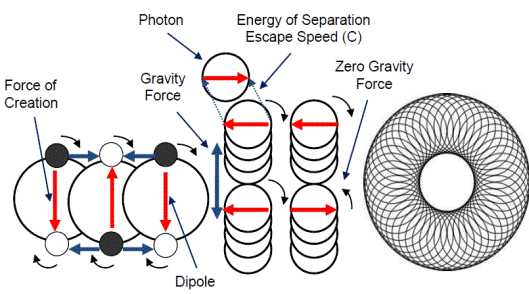Fig. 3. Wu’s Particle stack up in a preferred direction to form String and Ring Structures.

2.3. Electron and Electrical Force

When a multiple of "Wu’s Particle" come together, they can stack up to form String and Ring Structures such as that in Fig. 3, or overlap each other’s orbits to form a structure that is either with Yingtons circulating the Yangton Center such as the Electron or with Yangtons circulating the Yington Center as the Positron (Fig 4).

In Electron, because the repulsive force between Yangtons are smaller than the "Force of Creation", the attractive force between Yangton and Yington pair, Yangtons are loosely confined in the center of Electron, which is compressed by the centrifugal force induced from the circulation of Yingtons, therefore a sphere of Yingtons can be formed (Fig. 4). Similar mechanism can apply to Positron in which a sphere of Yangtons is structured (Fig. 4). Because of the “Force of Creation” between Yangton and Yington, a strong attractive force can be generated between Electron and Positron; and repulsive force between two Electrons also two Positrons. Since Positron is the anti-matter of Electron, they collide and destroy each other to release Gamma Ray (γ), this is known as Positron-Electron Annihilation.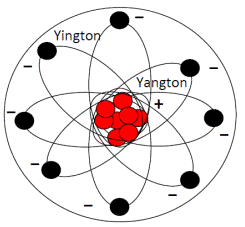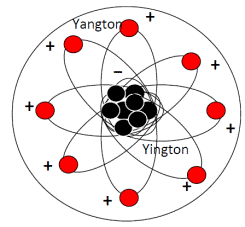Electron                                                                                  Positron

Fig. 4. Hypothetical Structures of Electron and Positron.

3. Higgs Boson and Gravitational Force

A Higgs Boson with String Structure made of "Wu’s Particle" has strong dipoles at both ends (Fig. 3). When two Higgs Bosons come together with the same circulation direction, attractive force can be generated between the contact points either at the ends or on the sides of the String Structures. But there is no interference for those with the opposite directions (Fig. 5). This attractive force is known as the Gravitational Force which is commonly found in the universe between substances.Fig. 5. Gravitational force between two Higgs Bosons.

4. Particle Radiation and Interaction Theory

Both Newton’s Law of Universal Gravitation and Coulomb’s Law of Electrical Forces only describe the phenomena without explanations of how they work. It is the purpose of this paper to propose a "Particle Radiation and Interaction Theory" to explain the mechanisms and the processes of the phenomena.

In a spherical "Particle Radiation" process, the concentration of emitted particles at any time interval should be proportional to the total amount of the particles in the parent substance. Along with the propagation process, the concentration of emitted particles will be diluted by a factor that is inversely proportional to the square of the distance from the parent substance as illustrated in Fig. 6. This is known as "Inverse Square Law".

It is believed that during the propagation process there is no interaction or interference happened, not until the end target substance is reached and “Contact Interaction” is occurred. The Contact Interaction can be selective. In case of Gravitational Force, since the Higgs Bosons in the target substance can have an angel anywhere from 0° to 180° away from the individual incoming Higgs Boson, only those Higgs Bosons in the target substance that is in parallel or partially parallel to the incoming Higgs Boson will make "Contact Interaction" and contribute to the attractive force at the contact point which is known as Gravitational Force (Fig. 6).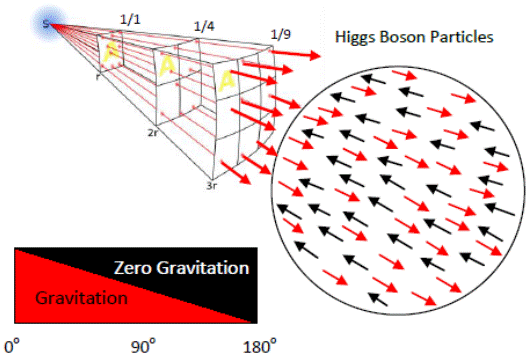Fig. 6. Inverse Square Law and Contact Interaction between Higgs Bosons having the same circulation direction.

4.1. Newton’s Law Based on Higgs Boson Radiation and Interaction Theory

Like Photons emitted spherically from a substance by absorbing thermal energy to overcome its "Energy of Separation", it is believed that Higgs Bosons with String Structures can also be emitted spherically from a substance by absorbing thermal energy to overcome its Gravitational Force. It is obvious that the concentration of the emitted Higgs Bosons should be proportional to the total amount of the Higgs Bosons in the parent substance which is the mass of the parent substance (m1). Also according to the Inversed Square Law, the concentration of the emitted Higgs Bosons that reaches the target substance for Contact Interaction should be inversely proportional to the square of the distance (r) between parent substance and target substance. Furthermore, only those Higgs Bosons in the target substance that is in parallel or partially parallel to the incoming Higgs Boson will contribute to the Gravitational Force, which is 50% of the total amount of Higgs Bosons in the target source (m2). Therefore the total Gravitational Force generated at the contact at any time should be proportional to the concentration of the emitted Higgs Bosons from the parent substance that touches down on the surface of the target substance (m1/r2) and the total amount of the Higgs Bosons in the target substance (m2) (Fig. 6).Therefore a formula such as Newton’s Law of Universal Gravitation (Fig. 7) can be derived as follows:

F = G (m1m2 r-2)

Where G is the gravitational constant (6.674×10−11 N m2 kg-2).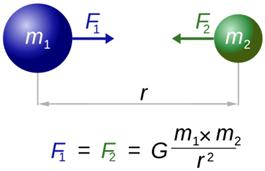Fig. 7. Gravitational force between two substances.

4.2. Coulomb’s Law Based on Electron Radiation and Interaction Theory

Similar to the Gravitational Force between two substances, at any time, the total amounts of the emitted Electrons from the parent charged particle that reaches the target charged particle causing Contact Interaction and producing either attraction or repulsion forces should be proportional to the amounts of charges in both parent charged particle (q1) and target charged particle (q2), also inversely proportional to square of distance (r) between the parent charged particle and the target charged particle. Therefore, a formula such as Coulomb’s Law of Electrical Forces (Fig. 8) can also be derived as follows:

|F| = ke (|q1 q2| r-2)

Where ke is Coulomb's constant (8.99×109 N m2 C−2). If the product q1q2 is positive, the force between the two charges is repulsive; if the product is negative, the force between them is attractive.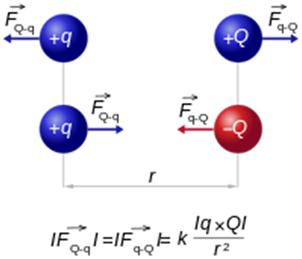Fig. 8. Electrical Force between two charged particles.

However, Electron Radiation from a negatively charged particle only happens in a much smaller scale than that of the Higgs Boson Radiation because that the negative charges (free electrons) are not only mobile, but also they are repulsive to each other and can be easily neutralized by the positive charges.

5. Gravitational Wave

Gravitational waves are proposed as 'ripples' in space-time which propagate like waves, travelling outward from the source caused by some of the most violent and energetic processes in the Universe. Albert Einstein predicted the existence of gravitational waves in 1916 in his general theory of relativity  . Einstein's mathematics showed that massive accelerating objects would disrupt space-time in such a way that 'waves' of distorted space would radiate from the source. Furthermore, these ripples would travel at the speed of light through the Universe, carrying with them information about their cataclysmic origins, as well as invaluable clues to the nature of gravity itself. By contrast, gravitational waves cannot exist in the Newton’s theory of gravitation, since Newton’s theory postulates that physical interactions propagate at infinite speed.

Potential sources of detectable gravitational waves include binary star systems composed of white dwarfs, neutron stars, stellar cores (supernovae) or black holes. On February 11, 2016, the LIGO Scientific Collaboration and Virgo Collaboration teams announced that they had made first observation of gravitational waves, originating from a pair of merging black holes using the Advanced LIGO detectors   .

6. Fluctuation of Gravitational Forces

According to the proposed “Particle Radiation and Interaction Theory”, the Gravitational Waves is actually the fluctuation of the total Gravitational Forces of the two merging stars, likes that of the total brightness (Fig. 9), Gravitational Waves is caused by one star blocking the other star detected in line of sight by the Earth observers. However, unlike that of the brightness, the fluctuation of the Gravitational Forces is very small and hard to detect except those caused by massive binary star rotation systems such as a pair of merging black holes.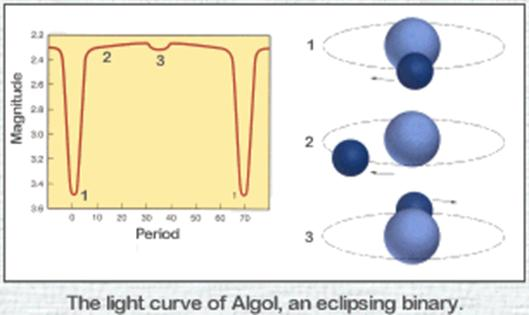Fig. 9. The Brightness of the eclipsing "Algol" binary system.

7. Conclusion

In conclusion, the structures of Photon, Higgs Boson, Electron, and Positron are proposed based on "Wu’s Particle", a Yangton and Yington circulating pair. It is believed that Gravitational Force is generated by the attractive force through close contact between two Higgs Bosons of String Structures made of "Wu’s Particle" having the same circulation direction. Through the propagation and interaction processes, Higgs Boson and Electron Radiations with Inverse Square Law and Contact Interaction, together defined as “Particle Radiation and Interaction Theory”, are proposed as the mechanisms of Newton’s Law of Universal Gravitation and Coulomb’s Law of Electrical Force. Furthermore, according to “Higgs Boson Radiation and Interaction Theory”, Gravitational Waves, instead of ripples of space-time, it is formed because of the fluctuation of the total Gravitational Forces from a pair of merging black holes detected in line of sight by Earth observers.

References

1. Edward T. H. Wu, "Yangton and Yington – A Hypothetical Theory of Everything", Science Journal of Physics, Volume 2015, Article ID sjp-242, 6 Pages, 2015, doi: 10.7237/sjp/242.
2. "Big-bang model". Encyclopedia Britannica. Retrieved 11 February 2015.
3. http://en.wikipedia.org/wiki/Photon.
4. Braibant, S.; Giacomelli, G.; Spurio, M. (2009). Particles and Fundamental Interactions: An Introduction to Particle Physics. Springer. pp. 313–314. ISBN 978-94-007-2463-1.
5. Walter Greiner (2001). Quantum Mechanics: An Introduction. Springer. p. 29.ISBN 3-540-67458-6.
6. Edward T. H. Wu. Subatomic Particle Structures and Unified Field Theory Based on Yangton and Yington Hypothetical Theory. American Journal of Modern Physics. Vol. 4, No. 4, 2015, pp. 189-195. doi: 10.11648/j.ajmp.20150404.15.
7. Davies, Paul (1986), The Forces of Nature, Cambridge Univ. Press 2nd ed.
8. Chandrasekhar, Subrahmanyan (2003). Newton's Principia for the common reader. Oxford: Oxford University Press. (pp.1–2).
9. Polchinski, Joseph (1998). String Theory, Cambridge University Press ISBN 0521672295.
10. Coulomb (1785a) "Premier mémoire sur l’électricité et le magnétisme,"Histoire de l’Académie Royale des Sciences, pages 569-577.
11. Ravaioli, Fawwaz T. Ulaby, Eric Michielssen, Umberto (2010). Fundamentals of applied electromagnetics (6th ed.). Boston: Prentice Hall. p. 13. ISBN 978-0-13-213931-1.
12. Purcell, E. (2011). Electricity and Magnetism (2nd ed.). Cambridge University Press. pp. 173–4. ISBN 1107013607.
13. B.P. Abbott et al. (LIGO Scientific Collaboration and Virgo Collaboration) (2016)."Observation of Gravitational Waves from a Binary Black Hole Merger".Physical Review Letters116 (6). Bibcode:2016PhRvL.116f1102A.doi:10.1103/PhysRevLett.116.061102.
14. Einstein, A (June 1916)."Näherungsweise Integration der Feldgleichungen der Gravitation". Sitzungsberichte der Königlich Preussischen Akademie der Wissenschaften Berlin. part 1: 688–696.
15. Einstein, A (1918)."Über Gravitationswellen". Sitzungsberichte der Königlich Preussischen Akademie der Wissenschaften Berlin. part 1: 154–167.
16. Castelvecchi, Davide; Witze, Witze (February 11, 2016)."Einstein's gravitational waves found at last". Nature News.doi:10.1038/nature.2016.19361. Retrieved 2016-02-11.
17. "Gravitational waves detected 100 years after Einstein's prediction | NSF - National Science Foundation". www.nsf.gov. Retrieved 2016-02-11.

 Contents 1. 2. 2.1. 2.2. 2.3. 3. 4. 4.1. 4.2. 5. 6. 7.
Article ToolsAbstractPDF(1071K)